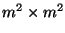Next: Weighted covariance methods Up: Algorithms for ICA Previous: The FastICA algorithm

## Tensor-based algorithms

A large amount of research has been done on algorithms utilizing the fourth-order cumulant tensor (see Section 4.3.5) for estimation of ICA [20,21,22,29,27,36]. These are typically batch algorithms (non-adaptive), using such tensorial techniques as eigenmatrix decomposition, which is a generalization of eigenvalue decomposition for higher-order tensors. Such a decomposition can be performed using ordinary algorithms for eigen-value decomposition of matrices, but this requires matrices of size. Since such matrices is often too large, specialized Lanczos type algorithms of lower complexity have also been developed . These algorithms often perform very efficiently on small dimensions. However, in large dimensions, the memory requirements may be prohibitive, because often the coefficients of the 4-th order tensor must be stored in memory, which requires O(m4) units of memory. The algorithms also tend to be quite complicated to program, requiring sophisticated matrix manipulations.

Aapo Hyvarinen
1999-04-23